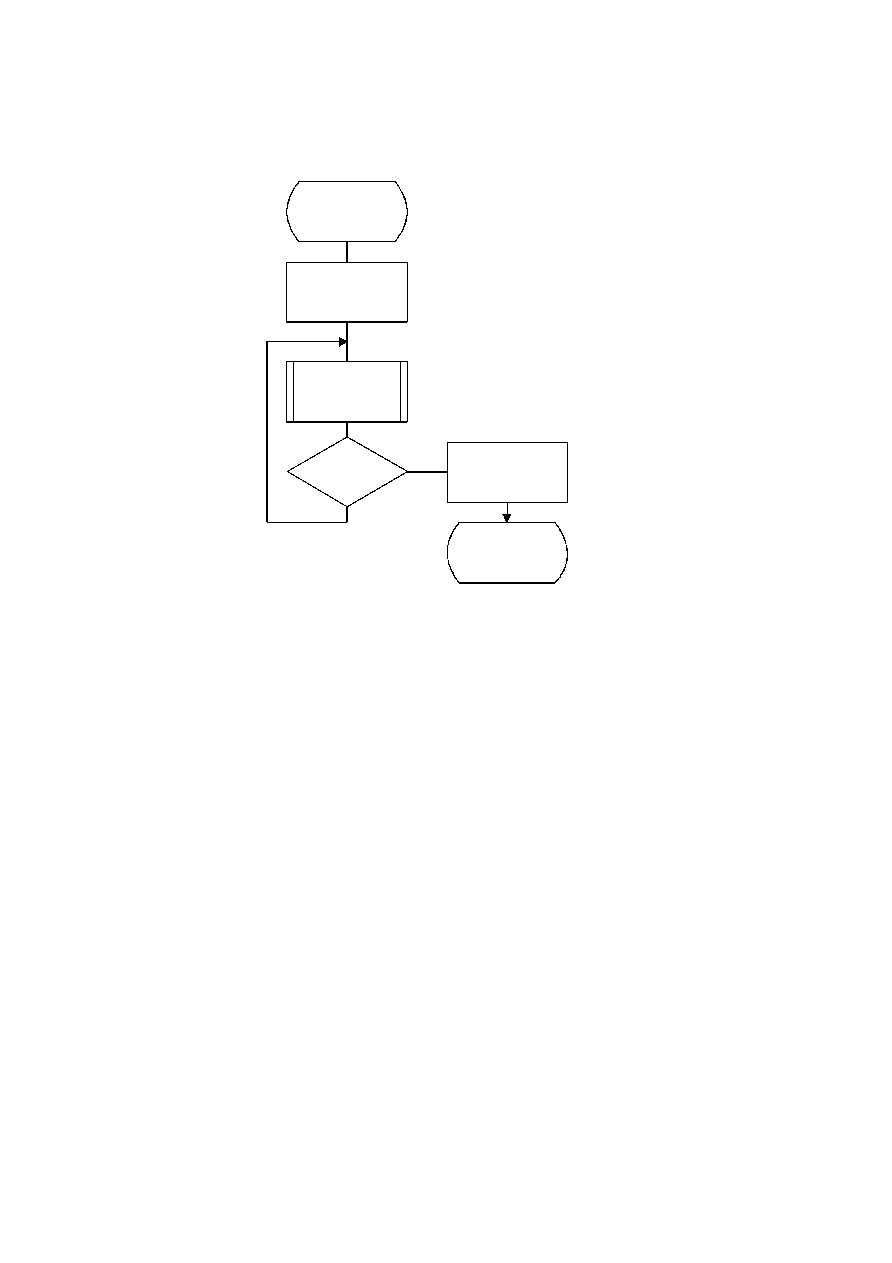ISO/IEC 10918-1 : 1993(E)
E.1.4
Control procedure for encoding a restart interval
Figure E.4 shows the encoding process control procedure for a restart interval. The loop is terminated either when the
encoding process has coded the number of minimum coded units (MCU) in the restart interval or when it has completed
the image scan.
TISO1260-93/d064
Reset_encoder
Encode_MCU
Prepare_for_marker
Done
Yes
No
Encode_restart_
interval
More MCU
?
Figure E.4 ­ Control procedure for encoding a restart interval
Figure E.4 [D64], = 12 cm = 469.%
The "Reset_encoder" procedure consists at least of the following:
a)
if arithmetic coding is used, initialize the arithmetic encoder using the "Initenc" procedure described
in D.1.7;
b)
for DCT-based processes, set the DC prediction (PRED) to zero for all components in the scan
(see F.1.1.5.1);
c)
for lossless processes, reset the prediction to a default value for all components in the scan (see H.1.1);
d)
do all other implementation-dependent setups that may be necessary.
The procedure "Prepare_for_marker" terminates the entropy-coded segment by:
a)
padding a Huffman entropy-coded segment with 1-bits to complete the final byte (and if needed stuffing a
zero byte) (see F.1.2.3); or
b)
invoking the procedure "Flush" (see D.1.8) to terminate an arithmetic entropy-coded segment.
NOTE ­ The number of minimum coded units (MCU) in the final restart interval must be adjusted to match the number
of MCU in the scan. The number of MCU is calculated from the frame and scan parameters. (See Annex B.)
80
CCITT Rec. T.81 (1992 E)